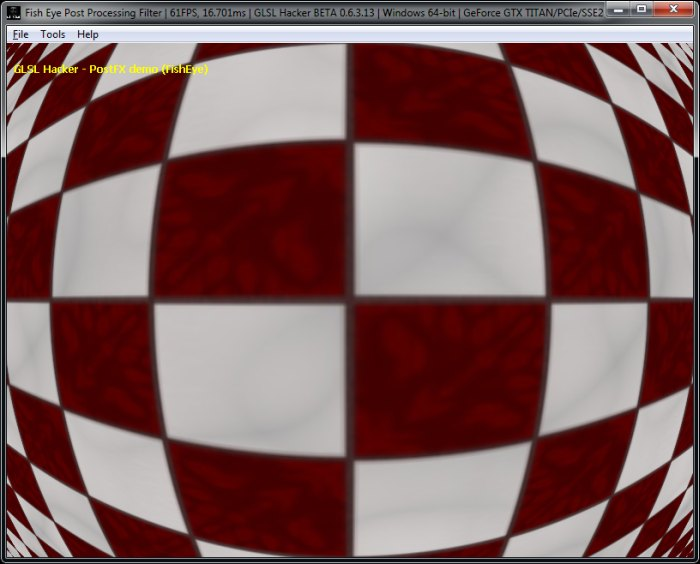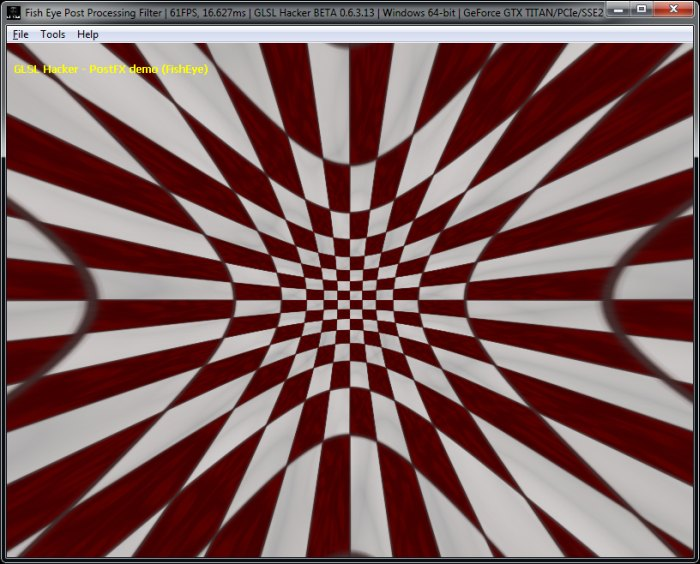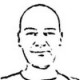# (Shader Library) Fish Eye, Dome and Barrel Distortion GLSL Post Processing Filters

Article index

This is another variation of the fish eye effect, this time from a Shadertoy demo:

```#version 120
uniform mat4 gxl3d_ModelViewProjectionMatrix;
void main()
{
gl_Position = gxl3d_ModelViewProjectionMatrix * gl_Vertex;
}
```

```#version 120
uniform sampler2D iChannel0;
uniform vec2 iResolution;
uniform vec2 iMouse;

#define EPSILON 0.000011

void main(void)//Drag mouse over rendering area
{
//normalized coords with some cheat
vec2 p = gl_FragCoord.xy / iResolution.x;

//screen proportion
float prop = iResolution.x / iResolution.y;
//center coords
vec2 m = vec2(0.5, 0.5 / prop);
//vector from center to current fragment
vec2 d = p - m;
// distance of pixel from center
float r = sqrt(dot(d, d));
//amount of effect
float power = ( 2.0 * 3.141592 / (2.0 * sqrt(dot(m, m))) ) *
(iMouse.x / iResolution.x - 0.5);
float bind;
if (power > 0.0) bind = sqrt(dot(m, m));//stick to corners
else {if (prop < 1.0) bind = m.x; else bind = m.y;}//stick to borders

//Weird formulas
vec2 uv;
if (power > 0.0)//fisheye
uv = m + normalize(d) * tan(r * power) * bind / tan( bind * power);
else if (power < 0.0)//antifisheye
uv = m + normalize(d) * atan(r * -power * 10.0) * bind / atan(-power * bind * 10.0);
else
uv = p;//no effect for power = 1.0

//Second part of cheat
//for round effect, not elliptical
vec3 col = texture2D(iChannel0, vec2(uv.x, -uv.y * prop)).xyz;

gl_FragColor = vec4(col, 1.0);
}
```1.fenbf